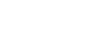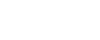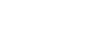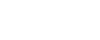### Calculus

* Not currently offered online with Maths Australia. Order directly on our shop page to have your materials shipped to you.#### THE CALCULUS LEVEL FOCUS:Derivatives, integrals, calculus applications, differential equations, and other topics

##### Major Concepts and Skills:
• Terminology and Graphing
• Review of Conic Sections
• Review of Systems of Equations
• Functions
• Review of Trigonometry
• Review of Exponents and Logarithms
• Limits
• Continuity in Limits
• Definition of a Derivative
• & more …
##### Purchase Calculus Complete Set
• Calculus Student Kit
• Calculus Instruction Manual
• Calculus Lesson-by-Lesson Instruction DVDs### \$259.00

+ GST

\$259.00 ex. GST#### Express shipped to youCalculus Instruction Manual:
1 x Pre Calculus Instruction Manual (with Solutions)

This manual will provide you with step-by-step instructions on how to teach each lesson to your student. This manual should be used in collaboration with the video lessons.Calculus Student Kit:
1 X Calculus Student Textbook
1 X Calculus Test Booklet
1 X Calculus Honors Booklet
1 X Calculus Student Completion Certificate

This workbook gives your student extra practice on what they've been taught from the Instruction manual and video lessons. The Honors booklet can be used as further practice for your student.Calculus Lesson-by-Lesson Instruction DVDs
2 X Calculus Instruction DVDs.
These lesson videos give you a step-by-step guide to teach the Math-U-See program using the correct methodology for maths mastery. The videos can be used to solidify the concepts in the instruction manual.##### The Calculus Complete Set includes:
• Calculus Student Kit
• Calculus Instruction Manual
• Calculus Lesson-by-Lesson Instruction DVDs

### \$259.00

+ GST

\$259.00 ex. GST##### Want to purchase your level materials separately?Calculus Instruction Manual
+ DVD Video Access

### \$199.00

+ GST

\$199.00 ex. GSTCalculus Student Kit

+ GST

\$79.00 ex. GST